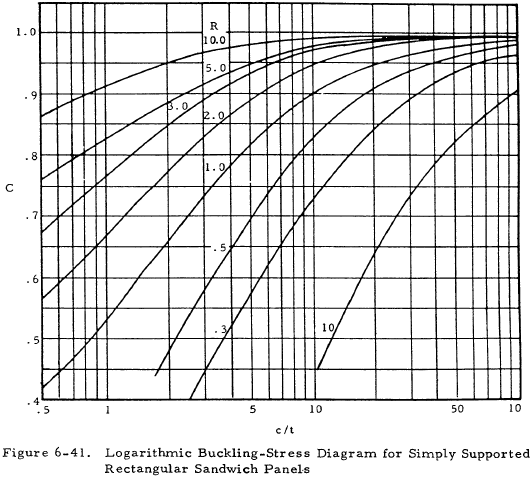﻿ Analysis of Plates - Special Topics | Engineering Library

# Analysis of Plates - Special Topics

This page provides the chapters on plates under combined loadings, buckling of oblique plates, and buckling of sandwich panels from the "Stress Analysis Manual," Air Force Flight Dynamics Laboratory, October 1986.

Other related chapters from the Air Force "Stress Analysis Manual" can be seen to the right.

## Nomenclature

 a = plate length b = plate width c = core thickness, signifies clamped edge C = compressive buckling coefficient for curved plates E = modulus of elasticity F = stress Fcr = critical normal stress Fcrs = critical shear stress Fcy = compressive yield stress
 kc = compressive buckling coefficient K = sandwich panel form factor R = stress ratio t = thickness Zb = length range parameter b2(1 - νe2)1/2/ rt η = plasticity reduction factor η = cladding reduction factor ν = inelastic Poisson's ratio

$$R_1^{~x} + R_2^{~y} = 1$$
(6-36)

Table 6-12 gives the combined loading condition for flat plates. Figures 6-36 and 6-37 give interaction curves for several loading and support conditions. It is noted that the curves present conditions of triple combinations.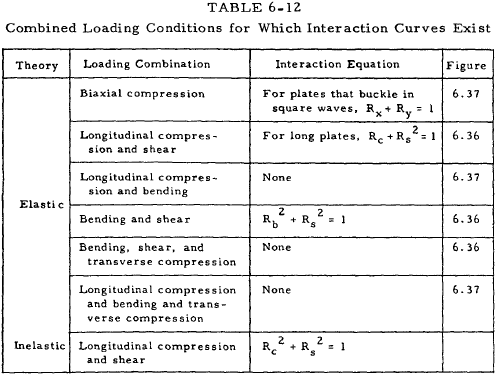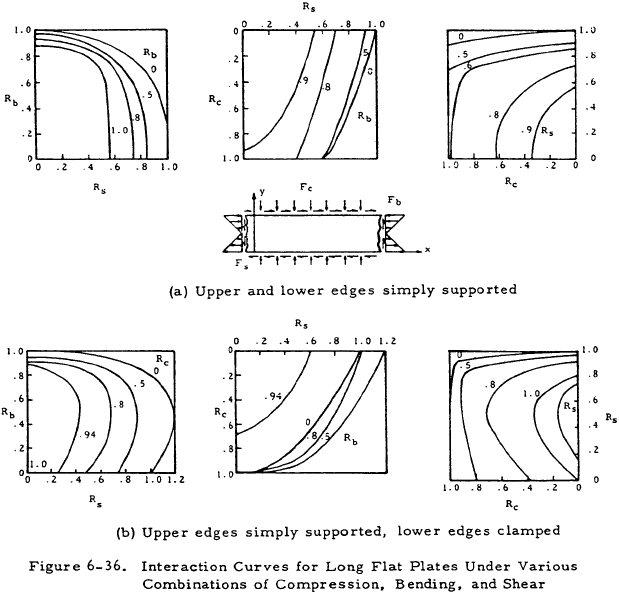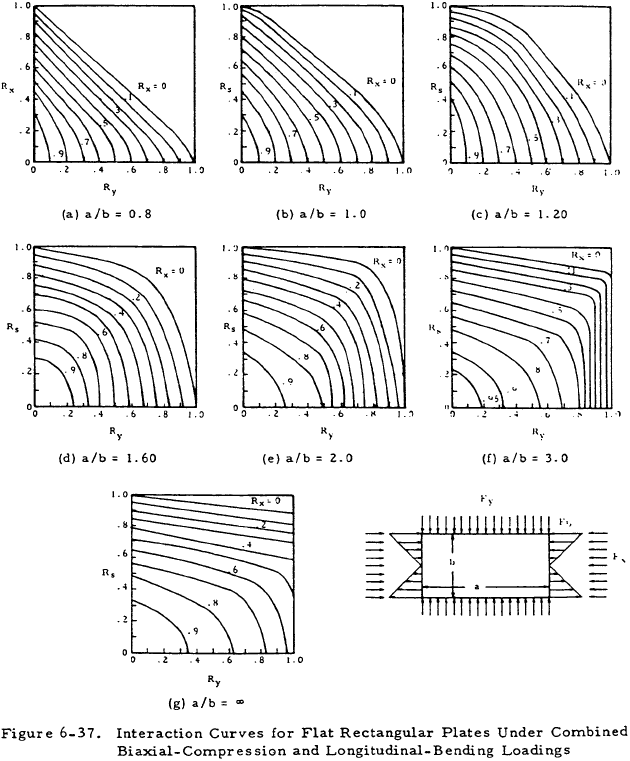Figure 6-38 presents buckling coefficients for right angle isosceles triangular plates loaded under shear and compression. Equation (6-37) is the interaction equation for shear and normal stress on this type of plate.

$$\left( { 2 ~F_s \over F_{crs+} + F_{crs-} } + u \right)^2 + { F \over F_{cr} } (1 - u^2) = 1$$
(6-37)

The + and − subscripts refer to either tension or compression along the altitude upon the hypotenuse of the triangle caused by pure shear loading. Table 6-13 contains values of kc, ks+ and ks− for various edge supports.

Table 6-13: Buckling Coefficients for Right-Angle Isosceles Triangular Plates Loaded Independently in Uniform Compression, Positive Shear, and Negative Shear
Edge Supports (Note a) kc ks+ ks−
All edges simply supported 10.0 62.0 23.2
Sides simply supported,
hypotenuse clamped
15.6 70.8 34.0
Sides clamped, hypottenuse
simply supported
18.8 80.0 44.0
Note a:  Hypotenuse = b in Figure 6.38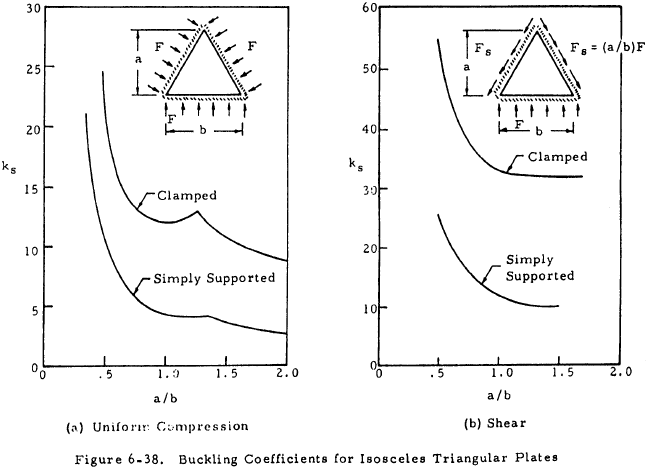For curved plates under combined axial loading and shear with 10 < Zb < 100 and 1 < a/b < 3, the interaction relation of Equation (6-39) may be used.

$$R_s^{~2} + R_x = 1$$
(6-39)

This may be used for either compression or tension with tension being denoted by a negative sign.

We have a number of structural calculators to choose from. Here are just a few:

## 6.9 Buckling of Oblique Plates

In many instances, the use of rectangular panels is not possible. Figures 6-39 and 6-40 give buckling coefficients for panels which are oriented oblique to the loading. Figure 6-39 covers flat plates divided into oblique parallelogram panels by nondeflecting supports. Figure 6-40 covers single oblique panels.## 6.10 Sample Problem - Plate Analysis

Find: The buckling stress of a flat plate under uniform longitudinal compression, simply supported on all four sides.

Givena = 12 in, b = 4 in, t = 0.100 in.

Material:  Bare 2024-T3 Sheet

 Material Properties: E = 10.7 x 106 psi ν = 0.33 Fcy = 34,000 psi

Solution:

$$F_{cr} = \overline{\eta} ~\eta ~{ k_c ~\pi^2 E \over 12 (1 - \nu^2) } \left({t \over b}\right)^2$$

From Figure 6-1 for a/b = 3, kc= 4.00.

 η = 1.0 for no cladding η = 1.0 for elastic buckling
$$F_{cr} = { (4.0) (\pi^2) (10.7) 10^6 \over 12 [1 - (0.33)^2] } \left({100 \over 2}\right)^2$$
 Fcr = 24,600 psi

As this is below the compression yield strength, no allowance for elasticity need be made.

We have a number of structural calculators to choose from. Here are just a few:

## 6.11 Buckling of Sandwich Panels

The use of sandwich construction for skin panels of aircraft has become commonplace. Panel construction consists of primary and secondary skins separated by a core which is usually some form of honeycomb or expanded foam material. The critical buckling stress of such a composite can be calculated from Equation (6-40).

$$F_{cr} = C ~K ~F_{crf}$$
(6-40)

The quantity Fcrf is the buckling stress of the two faces if they were not connected by the core. Use the total thickness of the faces. The coefficient C is obtained from Figure 6-41 and K is a form factor given in Equation (6-41).

$$K = 1 + 3 \left( 1 + {c \over t} \right)$$
(6-41)

The data in Figure 6-41 is given as a family of curves of parameter R which is given in Equation (6-42).

$$R = { G_{core} \over K ~F_{crf} }$$
(6-42)